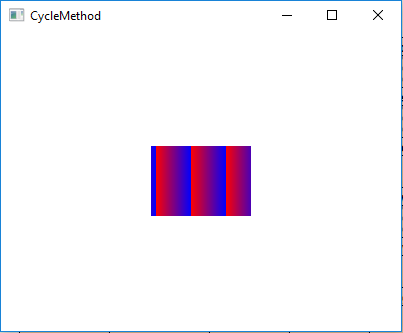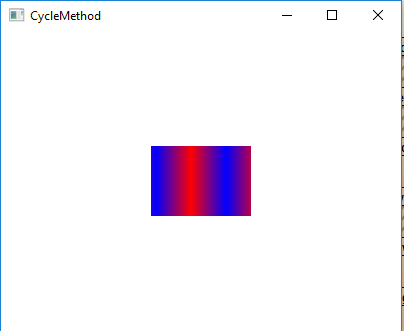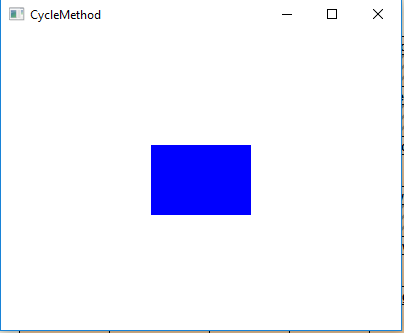# JavaFX | CycleMethod Class

CycleMethod class is a part of JavaFX. CycleMethod defines the method to use when painting outside the gradient bounds. It contains 3 Enum Constants as follows:

1. NO_CYCLE: Used to define the cycle method which uses terminal colors to fill the remaining area.
2. REFLECT: Used to define the cycle method which reflects the gradient colors, start to end then end to start.
3. REPEAT: Used to define the cycle method which repeats the gradient colors to fill the remaining area.

Commonly Used Method:

Method Explanation
valueOf(String name) Returns the CycleMethod of the specified name.
values() Returns an array which contains the values of Cyclemethod type.

Below programs illustrate the use of the CycleMethod class:

1. Java program to create a LinearGradient object, add stops to it, set the CycleMethod to repeat, set proportional to false and apply it to the rectangle:
1. In this program we will create an array of Stop objects with their offset values ranging from 0 to 1. Then create a LinearGradient object with specified stops.
2. Set the CycleMethod to repeat and set proportional to false. then create a circle with specified x, y positions, and radius and add the linear gradient to it.
3. After that create a VBox and set the alignment of it.
4. Add the circle to the vbox and add the vbox to the scene and add the scene to the stage and call the show() function to display the results.

 `// Java program to create a LinearGradient object, ` `// add stops to it, set the CycleMethod to repeat, ` `// set proportional to false and apply it to the ` `// rectangle ` `import` `javafx.application.Application; ` `import` `javafx.scene.Scene; ` `import` `javafx.scene.control.*; ` `import` `javafx.scene.layout.*; ` `import` `javafx.stage.Stage; ` `import` `javafx.scene.layout.*; ` `import` `javafx.scene.paint.*; ` `import` `javafx.scene.text.*; ` `import` `javafx.geometry.*; ` `import` `javafx.scene.layout.*; ` `import` `javafx.scene.shape.*; ` `import` `javafx.scene.paint.*; ` ` `  `public` `class` `CycleMethod_1 ``extends` `Application { ` ` `  `    ``// launch the application ` `    ``public` `void` `start(Stage stage) ` `    ``{ ` ` `  `        ``try` `{ ` ` `  `            ``// set title for the stage ` `            ``stage.setTitle(``"CycleMethod"``); ` ` `  `            ``// create stops ` `            ``Stop[] stop = {``new` `Stop(``0``, Color.RED),  ` `                         ``new` `Stop(``1``, Color.BLUE)}; ` ` `  `            ``// create a Linear gradient object ` `            ``LinearGradient linear_gradient = ``new` `LinearGradient(``0``, ``0``, ` `                             ``35``, ``0``, ``false``, CycleMethod.REPEAT, stop); ` ` `  `            ``// create a rectangle ` `            ``Rectangle rectangle = ``new` `Rectangle(``100``, ``100``, ``100``, ``70``); ` ` `  `            ``// set fill ` `            ``rectangle.setFill(linear_gradient); ` ` `  `            ``// create VBox ` `            ``VBox vbox = ``new` `VBox(rectangle); ` ` `  `            ``// ste Alignment ` `            ``vbox.setAlignment(Pos.CENTER); ` ` `  `            ``// create a scene ` `            ``Scene scene = ``new` `Scene(vbox, ``400``, ``300``); ` ` `  `            ``// set the scene ` `            ``stage.setScene(scene); ` ` `  `            ``stage.show(); ` `        ``} ` ` `  `        ``catch` `(Exception e) { ` ` `  `            ``System.out.println(e.getMessage()); ` `        ``} ` `    ``} ` ` `  `    ``// Main Method ` `    ``public` `static` `void` `main(String args[]) ` `    ``{ ` ` `  `        ``// launch the application ` `        ``launch(args); ` `    ``} ` `} `

Output:2. Java program to create a LinearGradient object, add stops to it, set the CycleMethod to reflect, set proportional to false and apply it to the circle:
1. In this program we will create an array of Stop objects with their offset values ranging from 0 to 1.
2. Then create a LinearGradient object with specified stops. Set the CycleMethod to reflect and set proportional to false.
3. Create a circle with specified x, y positions, and radius and add the linear gradient to it. Create a VBox and set the alignment of it.
4. Add the circle to the vbox and add the vbox to the scene and add the scene to the stage.
5. Call the show() function to display the results.

 `// Java program to create a LinearGradient object, ` `// add stops to it, set the CycleMethod to reflect, ` `// set proportional to false and apply it to the  ` `// circle ` `import` `javafx.application.Application; ` `import` `javafx.scene.Scene; ` `import` `javafx.scene.control.*; ` `import` `javafx.scene.layout.*; ` `import` `javafx.stage.Stage; ` `import` `javafx.scene.layout.*; ` `import` `javafx.scene.paint.*; ` `import` `javafx.scene.text.*; ` `import` `javafx.geometry.*; ` `import` `javafx.scene.layout.*; ` `import` `javafx.scene.shape.*; ` `import` `javafx.scene.paint.*; ` ` `  `public` `class` `CycleMethod_2 ``extends` `Application { ` ` `  `    ``// launch the application ` `    ``public` `void` `start(Stage stage) ` `    ``{ ` ` `  `        ``try` `{ ` ` `  `            ``// set title for the stage ` `            ``stage.setTitle(``"CycleMethod"``); ` ` `  `            ``// create stops ` `            ``Stop[] stop = {``new` `Stop(``0``, Color.RED), ` `                         ``new` `Stop(``1``, Color.BLUE)}; ` ` `  `            ``// create a Linear gradient object ` `            ``LinearGradient linear_gradient = ``new` `LinearGradient(``0``, ``0``,  ` `                            ``35``, ``0``, ``false``, CycleMethod.REFLECT, stop); ` ` `  `            ``// create a rectangle ` `            ``Rectangle rectangle = ``new` `Rectangle(``100``, ``100``, ``100``, ``70``); ` ` `  `            ``// set fill ` `            ``rectangle.setFill(linear_gradient); ` ` `  `            ``// create VBox ` `            ``VBox vbox = ``new` `VBox(rectangle); ` ` `  `            ``// ste Alignment ` `            ``vbox.setAlignment(Pos.CENTER); ` ` `  `            ``// create a scene ` `            ``Scene scene = ``new` `Scene(vbox, ``400``, ``300``); ` ` `  `            ``// set the scene ` `            ``stage.setScene(scene); ` ` `  `            ``stage.show(); ` `        ``} ` ` `  `        ``catch` `(Exception e) { ` ` `  `            ``System.out.println(e.getMessage()); ` `        ``} ` `    ``} ` ` `  `    ``// Main Method ` `    ``public` `static` `void` `main(String args[]) ` `    ``{ ` ` `  `        ``// launch the application ` `        ``launch(args); ` `    ``} ` `} `

Output:3. Java program to create LinearGradient object, add stops to it, set the CycleMethod to no cycle, set proportional to false and apply it to the rectangle:
1. In this program we will create an array of Stop objects with their offset values ranging from 0 to 1.
2. Create a LinearGradient object with specified stops.
3. Set the CycleMethod to no cycle and set proportional to false. Then Create a circle with specified x, y positions, and radius, and add the linear gradient to it. Create a VBox and set the alignment of it.
4. Now add the circle to the vbox and add the vbox to the scene and add the scene to the stage and call the show() function to display the results.

 `// Java program to create LinearGradient object, ` `// add stops to it, set the CycleMethod to no  ` `// cycle, set proportional to false and apply  ` `// it to the rectangle ` `import` `javafx.application.Application; ` `import` `javafx.scene.Scene; ` `import` `javafx.scene.control.*; ` `import` `javafx.scene.layout.*; ` `import` `javafx.stage.Stage; ` `import` `javafx.scene.layout.*; ` `import` `javafx.scene.paint.*; ` `import` `javafx.scene.text.*; ` `import` `javafx.geometry.*; ` `import` `javafx.scene.layout.*; ` `import` `javafx.scene.shape.*; ` `import` `javafx.scene.paint.*; ` ` `  `public` `class` `CycleMethod_3 ``extends` `Application { ` ` `  `    ``// launch the application ` `    ``public` `void` `start(Stage stage) ` `    ``{ ` ` `  `        ``try` `{ ` ` `  `            ``// set title for the stage ` `            ``stage.setTitle(``"CycleMethod"``); ` ` `  `            ``// create stops ` `            ``Stop[] stop = {``new` `Stop(``0``, Color.RED), ` `                         ``new` `Stop(``1``, Color.BLUE)}; ` ` `  `            ``// create a Linear gradient object ` `            ``LinearGradient linear_gradient = ``new` `LinearGradient(``0``, ``0``,  ` `                           ``35``, ``0``, ``false``, CycleMethod.NO_CYCLE, stop); ` ` `  `            ``// create a rectangle ` `            ``Rectangle rectangle = ``new` `Rectangle(``100``, ``100``, ``100``, ``70``); ` ` `  `            ``// set fill ` `            ``rectangle.setFill(linear_gradient); ` ` `  `            ``// create VBox ` `            ``VBox vbox = ``new` `VBox(rectangle); ` ` `  `            ``// ste Alignment ` `            ``vbox.setAlignment(Pos.CENTER); ` ` `  `            ``// create a scene ` `            ``Scene scene = ``new` `Scene(vbox, ``400``, ``300``); ` ` `  `            ``// set the scene ` `            ``stage.setScene(scene); ` ` `  `            ``stage.show(); ` `        ``} ` ` `  `        ``catch` `(Exception e) { ` ` `  `            ``System.out.println(e.getMessage()); ` `        ``} ` `    ``} ` ` `  `    ``// Main Method ` `    ``public` `static` `void` `main(String args[]) ` `    ``{ ` ` `  `        ``// launch the application ` `        ``launch(args); ` `    ``} ` `} `

Output:Note: The above programs might not run in an online IDE please use an offline compiler.

Attention reader! Don’t stop learning now. Get hold of all the important DSA concepts with the DSA Self Paced Course at a student-friendly price and become industry ready.

My Personal Notes arrow_drop_upCheck out this Author's contributed articles.

If you like GeeksforGeeks and would like to contribute, you can also write an article using contribute.geeksforgeeks.org or mail your article to contribute@geeksforgeeks.org. See your article appearing on the GeeksforGeeks main page and help other Geeks.

Please Improve this article if you find anything incorrect by clicking on the "Improve Article" button below.

Article Tags :
Practice Tags :

Be the First to upvote.

Please write to us at contribute@geeksforgeeks.org to report any issue with the above content.